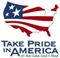Scientific Investigations Report 2007–5186

U.S. GEOLOGICAL SURVEY
Scientific Investigations Report 2007–5186

Table 3. Rules for computing total nitrogen concentration from total Kjeldahl nitrogen and dissolved nitrite plus nitrate as nitrogen.

[Concentrations are in milligrams per liter. A notation of “Value(nitrite plus nitrate) greater than or equal to (≥) 0.05” means that the concentration (value) of the combined sum of the nitrite plus nitrate species is greater than or equal to 0.05 milligrams per liter. 0.05 is the detection limit selected for nitrite plus nitrate, 0.025 is one-half this value; 0.2 is the detection limit for TKN, and 0.1 is one-half this value. Abbreviations: TN, total nitrogen; TKN, total Kjeldahl nitrogen; <, less than]

Case No. Nitrite plus nitrate TKN Condition TN
1 <0.05 <0.20 Nitrite plus nitrate is <0.05 and TKN is <0.20 <0.25
2 Value(nitrite plus nitrate) ≥ 0.05 Value(TKN) ≥ 0.2 Value(nitrite plus nitrate) ≥ 0.05 and  Value(TKN) ≥ 0.2 Value(nitrite plus nitrate) + Value(TKN)
3a <0.05 Value(TKN) ≥ 0.2 Value(TKN) + 0.025 < 0.25 <0.25
3b <0.05 Value(TKN) ≥ 0.2 Value(TKN) + 0.025 ≥ 0.25 Value(TKN) + 0.025
4a Value(nitrite plus nitrate) ≥ 0.05 <0.20 Value(nitrite plus nitrate) + 0.1 < 0.25 <0.25
4b Value(nitrite plus nitrate) ≥ 0.05 <0.20 Value(nitrite plus nitrate) + 0.1 ≥ 0.25 Value(nitrite plus nitrate) + 0.1
5 Missing Missing Nitrite plus nitrate is missing or TKN is missing MissingU.S. Department of the Interior | U.S. Geological Survey